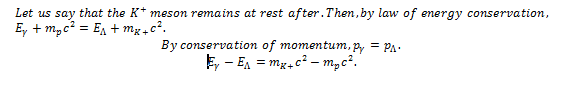# Special Relativity: Photoproduction of Mesons?

## Homework Statement

12-16 K + mesons can be photoproduced by the reaction γ + p → K+ + Λ where the initial proton is at rest in the lab. From the conservation laws, discover if it is possible for either the K+ or the Λ to be at rest in the lab, and for what photon energy (in terms of the particle masses) this could happen.

## Homework Equations

E^2 = p^2c^2 + m^2c^4
p = mv
E = mc^2

## The Attempt at a SolutionI'm really stuck on this problem. It's probably more of an algebraic answer, because I believe I'm supposed to somehow get the two equations of energy and momentum conservation to have like terms and then combine them somehow, but I'm at a loss as to what to do. Any help is appreciated.

Conservation of Momentum: $\displaystyle{p_\gamma =p_\Lambda \Rightarrow E_\gamma =p_\Lambda c\Rightarrow E_\gamma ^2=E_\Lambda ^2-m_\Lambda ^2c^4}$
If you combine this with the conservation of energy you have two equations and two uknowns, $\displaystyle{E_\gamma }$ and $\displaystyle{E_\Lambda}$.